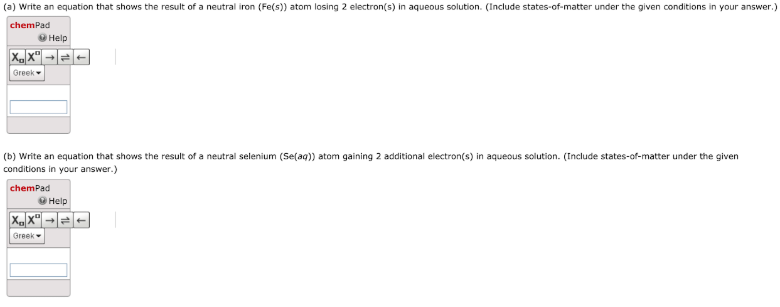# (a) Write an equation that shows the result of a neutral iron (Fe(s)) atom losing 2 electron(s) in aqueous solution. (Include states-of-matter under the given conditions in your answer. Use the lowest possible whole number coefficients.) (b) Write an equation that shows the result of a neutral selenium (Se(aq)) atom gaining 2 electron(s) in aqueous solution. (Include states-of-matter under the given conditions in your answer. Use the lowest possible whole number coefficients.)